# R Tutorial: a quick beginner's guide to using R

Aug 27, 2020 - 14 min read
Aaron Xie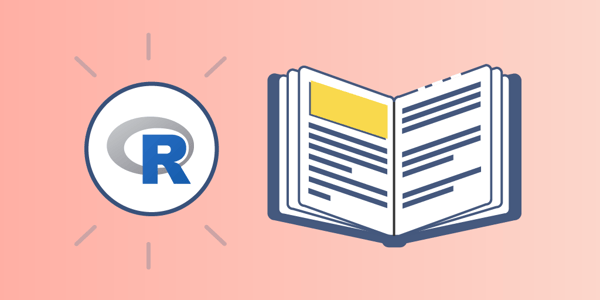The programming language R is incredibly popular. Whether you look at the 10,000 packages it offers or that it’s the sixth most popular programming language, there’s no doubt that R is rising in popularity. As fields like data science and machine learning grow, the use of R follows.

So, why use R? What even is the R programming language? Today, we’ll provide an introductory guide to the R programming language so you can start using this popular, versatile language.

Today, we’ll cover:

## A brief history of R

The R programming language is an implementation of the S programming language, which was created by John Chambers at Bell Labs.

R was created by Robert Gentleman and Ross Ihaka at the University of Auckland, New Zealand. The team combined S with lexical scoping semantics to create R. The R project was first conceived in 1992, and then initial released in 1995. On February 29, 2000, a stable beta version was released.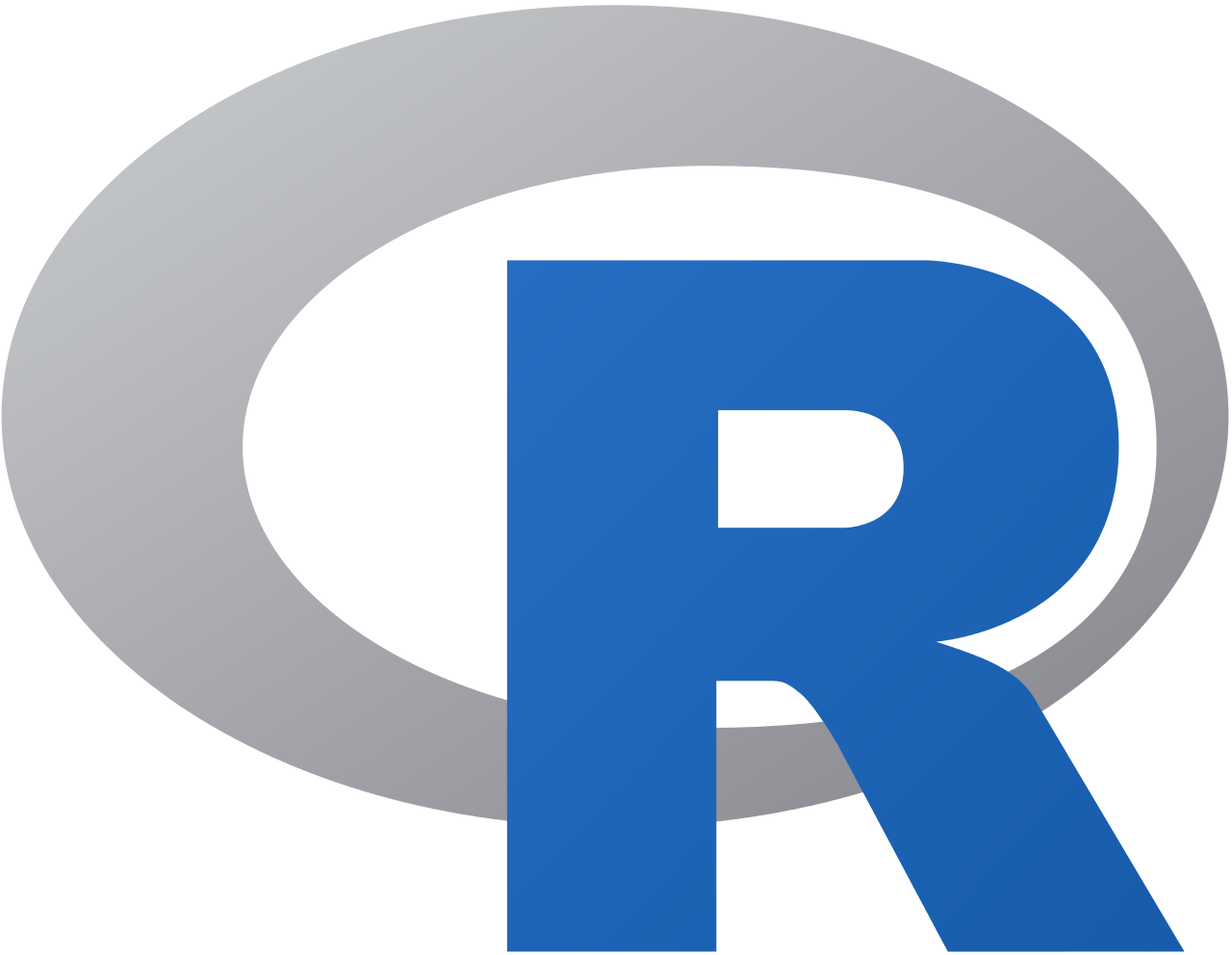## Overview of R

R is a programming language and environment used for statistical computing and graphics. R provides a large variety of statistical (linear and nonlinear modeling, classical statistical tests, clustering, time-series analysis, classification, etc.) and graphical techniques. It is also highly extensible.

Before, the S language was the popular choice for research in statistical methodology. When R was released, it was the open-source route to participate in this activity and has risen in popularity since then.

### Environment

R is a suite of software facilities and environment used for data manipulation, calculator, and graphs. Some of the features that it offers includes:

• A powerful data handling and storage facility
• A suite of operators that can be used for calculations on arrays and in particular matrices
• A large and integrated collection of tools used for data analysis
• Graphical facilities for data analysis and to display on screen or on a hardcopy
• A robust, comprehensive, simple programming language that includes conditionals, loops, user-defined recursive functions, and input/output facilities

The term “environment” is intentionally used to describe R as a system rather than simply a programming language. R is frequently used along with other data analysis tools.

### Why should you use R?

• Open-source and Free: R is free to download as licensed under the terms of the GNU General Public License. If you want to see what’s actually happening under the hood, you can look at the source code. Beyond that, you have access to a ton of R packages under the same license that you can use. You can use is in commercial applications as well.

• Popularity: The IEEE published a list of the most popular programming language, and it was 6th in 2020, which it has stabilized at around that rank. R is more popular than general purpose languages like C#, indicating the increased interest in the R programming language specifically, as well as the general growth in fields like data science and machine learning.

• Runs on all platforms: You can find distributions of R on all the popular platforms: Windows, Linux, and Mac. Furthermore, R code that is written on one platform can easily be translated to another platform with little to no issues. R’s cross-platform interoperability is incredibly important in today’s computing world, as seen by Microsoft seeking to make its .NET platform available on all platforms.

• Job market: Data scientists in the United States are being paid over $100,000 on average. Many data scientist roles require you to know the R programming language. Though knowing R won’t automatically get you a job, as data scientists are required to use all kinds of tools for their work, R programming experience will help you stand out among other applicants. • Tech giant adoption: If tech giants are adopting a programming language, that’s a sign of the language’s potential and growth. Due to R’s simplicity and power, companies are making calculated decisions to use the R programming language and environment. For example, Twitter uses R to monitor user experience, Ford to analyze social media, and New York Times for infographics.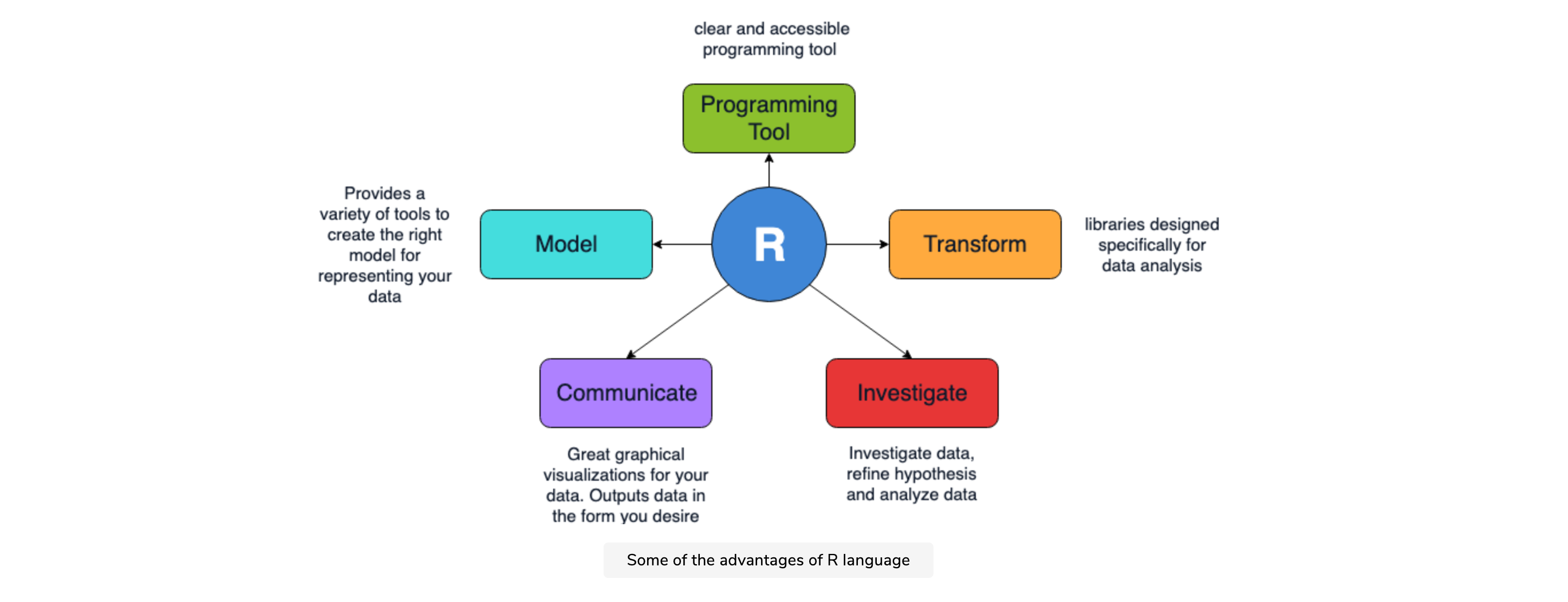### Is R difficult to learn? R is no more difficult than any other language, especially if you already have some experience with older languages like C or C++. Many years ago, most would have said that R was a difficult language to learn. Not only was it confusing, but it was also not structured well. To solve these issues, Hadley Wickham created a collection of packages called tidyverse, which made data manipulation more intuitive. Now, the best algorithms for machine learning can be implemented through R with ease. From packages to Keras to TensorFlow to Xgboost, you’ve given quite powerful functionality when using the R language. Beyond that, R has evolved to allow for parallelizing operation to speed up its computation. The package allows you to perform simultaneous tasks rather that only one. ## Real-world uses of R So, what are the main uses of R in the field of computer engineering? R is used for: • Statistical inference • Data analysis • Machine learning • Executing scientific simulations • Operations research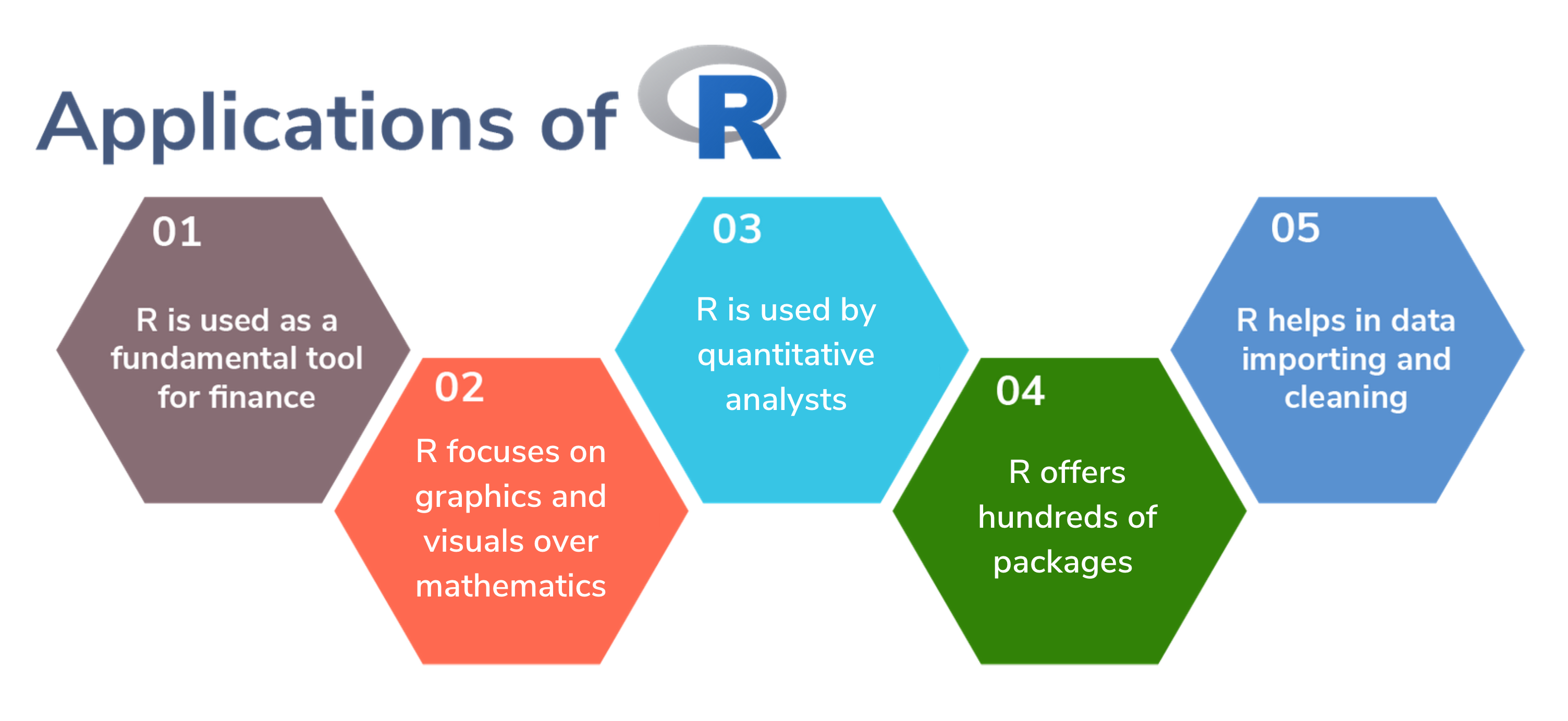### Statistical computing The R programming language was initially built for statisticians by statisticians. R is by far the most popular programming language used by statisticians. R’s syntax allows researches to easily import, clean, and analyze their data from a wide variety of sources. Beyond that, R offers wide and powerful capabilities for charting, meaning that you can plot data and create visualizations. ### Data science In many ways, a data scientist is a statistician with an additional skill: computer programming skills. R allows data scientists to collect data in real time, perform predictive and statistical forms of analysis, create visualizations, and also communicate results to necessary stakeholders. R is a favorite tool for data scientists. ### Machine learning (ML) R is commonly used in predictive analytics and ML. Some useful packages offered by the R ecosystem are linear and non-linear regression, decision trees, linear and non-linear classification, and more. R can implement ML algorithms in fields such as retail, marketing, finance, and more. ### Real-world example In just three lines of code, you’ll be able to generate 10,000 numbers in random distribution. That’s the power of R. If we write this code: n <- floor(rnorm(10000, 500, 100)) t <- table(n) barplot(t)  At the bottom right, you will be able to see this graph: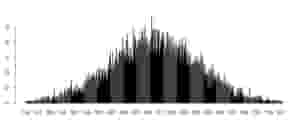• The first line in the code generates a list of 10,000 random numbers in a normal distribution pattern such that the mean of these numbers is 500 and the standard deviation is 100. The floor function takes all the numbers in the list and removes the decimal point. • For the second line of code, the table function takes the 10,000 numbers and counts the frequency of each. • In the third line of code, The barplot function takes this table of frequencies and creates the bar chart out of the data. ## R tools, packages, and syntax Now that we know more about R and its uses, let’s get started with the R syntax. This is the way that we actually write code in R to make our computer respond accordingly. We’ll also need to learn about the tools and packages necessary for writing in R. Let’s dive in. To be most successful with this section, some basic knowledge of programming terms is helpful. If you’re new, I recommend reading The absolute beginner’s guide to coding before continuing here. ### Workspace The workspace is your current working R environment, which includes user-designed objects such as matrices, vectors, data frames, lists, and functions. After your session, you can save an image of your current workspace, which will automatically be reloaded once you start R again. ### Graphic User Interface Aside from the built-in R console, RStudio is the most popular R editor, which can interface R with Windows, MacOS, and Linux platforms. ### Operators in R R’s operators look similar to other programming languages. Some arithmetic operators include: • + - addition • - - subtraction • * - multiplication • / - division • ^ - exponentiation Logical operators include: • > - greater than • >= - greater than or equal to • == - exactly equal to • != - not equal to ### Data types R has five main data types. In R language, if you change the data in a variable, then the previous information will be deleted. So, each data type needs a unique name.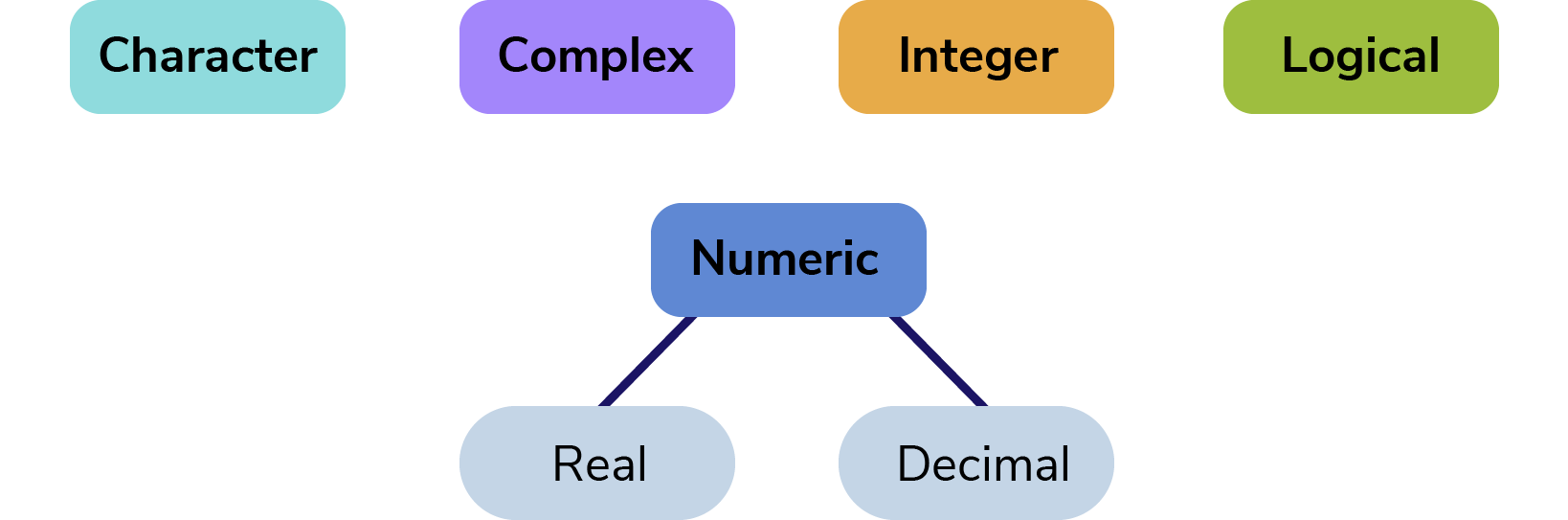## Get started with R for free. Take our introductory R course and expand your skills efficiently. Educative’s text-based courses are easy to skim and feature live coding environments - making learning quick and efficient. Learn R from Scratch ### Creating variables Variables are used to store data. Their value can be changed, used and manipulated according to need. A unique name given to a variable (function or object as well) is called an identifier. Note: In R, identifiers can have a combination of letters, digits, one period . and one underscore. However, they must start with a letter or a period. If it starts with a period, it cannot be followed by a digit. To declare a variable, we need to assign a variable an identifier. Use the <- assignment operator to create a new variable. # An example of computing the mean with variables mydata$sum <- mydata$x1 + mydata$x2
mydata$mean <- (mydata$x1 + mydata\$x2)/2

### Methods in R

Methods are like built-in operations that we can apply to our code. Let’s look at two popular methods to get familiar with how they work in R.

Listing variables

We can check all the variables that have been created in the workspace using the keyword ls(). Try it out below.

myRealNumeric <- 10
myDecimalNumeric <- 10.0
myCharacter <- "10"
myBoolean <- TRUE
myInteger <- 0:10
myComplex <- 5i

cat("Variables in the current directory: \n")
ls() # returns all the variables created in the workspace alphabetically
cat("\n")

Deleting variables

We can delete a specific variable from the workspace. The keyword rm() can help us permanently remove one or more objects from the workspace. Try it out below.

myRealNumeric <- 10
myDecimalNumeric <- 10.0
myCharacter <- "10"
myBoolean <- TRUE
myInteger <- 0:10
myComplex <- 5i

cat("Variables in the current directory: \n")
ls() # returns all the variables created in the workspace
cat("\n")

cat("Deleting myRealNumeric and myDecimalNumeric \n\n")

rm(myRealNumeric, myDecimalNumeric) # delete the two mentioned variables

cat("Variables in the current directory, now: \n")
ls() # returns all the variables created in the workspace
# myRealNumeric, myDecimalNumeric are now deleted
cat("\n")

### Functions

Essentially everything in R is done through functions. A function is a block of code written for a specific task or series of tasks. It can accept parameters and may return a value if defined. A function in R is defined below. The code in between the curly braces is the body of the function.

function ( arglist ) {body}

### Strings: print() and cat()

In R, we can express character strings by surrounding text with double quotes or single quotes. To write strings, we use the syntax cat(). We can also find the length of a string with the method nchar().

cat("Hello world\n")
nchar("Hello World")


You’ll notice our string ends with /n. A sequence that starts with a \ in a string is called an escape sequence. It allows us to include special characters in our strings. Common escape sequences are: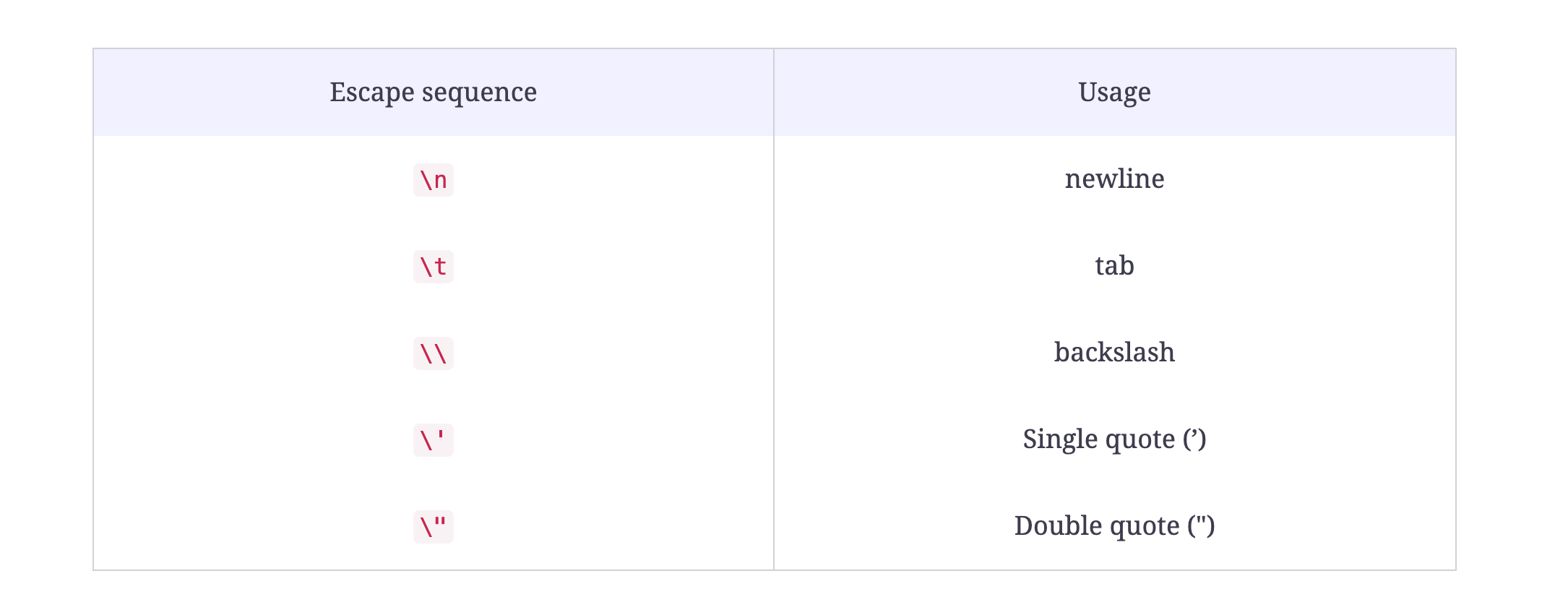We can also use the method print(), which may look familiar to you if you work with other languages. There is a slight differences between the two:

print() returns a character vector. A vector is an object in R language. cat() returns an object NULL. If you want to learn more about this, you should investigate atomic types in R.

But, at the most basics level: cat() prints its arguments without quotes, and print() will display them.

### Vectors

A Vector is a basic data structure in R. It contains elements of the same type at each index. The keyword vector() is used to create a vector of a fixed type and fixed length. The data types can be:

• Logical
• Integer
• Numeric
• Character
• Complex

A vector’s type can be checked with typeof(), and the number of elements in the vector can be checked with length().

vector ("numeric", 5) # numeric vector with O at every index

vector ("complex", 5) # complex vector with O+0i at every index

vector ("logical", 5) # logical vector with FALSE at every index

vector ("character", 5) # character vector with "" at every index

### Import data

Importing data in R is relatively easy, since R offers many options to import included CSV. This is an important feature for data science.

Below is an example of importing a CSV file to your R project.

# first row contains variable names, comma is separator
# assign the variable id to row names
# note the / instead of \ on mswindows systems

sep=",", row.names="id")

### Plotting in R

In R, graphics are created interactively, as seen below.

# Creating a Graph
attach(mtcars)
plot(wt, mpg)
abline(lm(mpg~wt))
title("Regression of MPG on Weight")

The plot() function allows you to open a graph window which will plot weight vs. miles per gallon. The next line adds a regression line to the graph. Finally, the last line adds a title to finish off.

### Packages

Packages are commonly used in the R programming language, with thousands in its collection. Packages contain a collection of R functions, data, and compiled code in a defined form. R comes with a standardized set of packages, with other packages available for download. You can load the packages in session, as seen below.

.libPaths() # get library location
library()   # see all packages installed
search()    # see packages currently loaded

### Getting help

When you install R, you will have access to a comprehensive built-in help system. You can use any of the following codes.

help.start()   # general help
help(foo)      # help about function foo
?foo           # same thing
apropos("foo") # list all functions containing string foo
example(foo)   # show an example of function foo

## Creating your first R project: Hello World

Now we know the basic syntax of R and the tools we need to use it. Let’s get hands-on with R and learn how to create an application with this language.

On Mac:

1. Go to the R site
2. Click on the CRAN link
3. Select a mirror
6. Run the file and follow the steps as you install R

On Windows:

1. Go to the R site
2. Click on the CRAN link
3. Select a mirror
6. Run the file and follow the steps as you install R

### Installing RStudio

As state above, RStudio is the most popular IDE for running R programs. You can download it here for Windows, Linux, and Mac OS.

R is known for being able to create applications with little code. Let’s try two projects, starting with Hello World. Try. it yourself before checking the solution. Remember: you can use cat or print().

# write your code here

As you can see, R is pretty simple! In fact, let’s try another program.

In this program, we want to use two separate cat() statements to display text on the screen, one for high level and a second for low-level.

• Our input is a testVariable containing the variable being tested.
• Our output is the high-level and low-level data types of that variable.
#input
1.9

# output
numeric
double


Let’s walk through it step-by-step. Firt, we have to define our variable.

testVariable <- 1.9


Now, to find the high-level data type of the given testVariable, wee use the class() keyword with the given testVariable. We pass this to the method cat() for printing and also add \n for a new line.

cat(class(testVariable), "\n")
# high level data type


A class is like a blueprint that helps to create an object and contains its member variable along with the attributes.

Now, we need to print the low-level data type of the testVariable and for that, we use typeof() with the testVariable.

cat(typeof(testVariable), "\n")
# low level of variable


Let’s put it all together and run our code!

# write your R code here

## What to learn next

Congratulations! You’ve learned the basics of R and written two programs! Now, you’re ready to start learning more complex concepts. The next steps to master R are as follows:

• Lists and arrays
• Matrices and data frames
• Operators and notation
• Conditional statements
• Exception handling
• and more

To get you started learning these advanced topics, Educative has created a free course, Learn R from Scratch. You’ll use hands-on activities and real-world examples to master R from scratch. By the end, you’ll be on your way to becoming a data scientist!

Happy learning!